# Multiplication and Division in Scientific Notation

##### Rating:
(3)
• (1)
• (1)
• (1)
• (0)
• (0)
Author: Sophia Tutorial
##### Description:

Multiply or divide numbers in scientific notation.

(more)
Tutorial
what's covered
1. Multiplying and Dividing with Scientific Notation

# 1. Multiplication and Division with Scientific Notation

When converting between standard notation and scientific notation it is important to understand how scientific notation works and what it does. Here our main interest is to be able to multiply and divide numbers in scientific notation using exponent properties. The way we do this is to first do the operation with the front number (multiply or divide) then use exponent properties to simplify the 10’s. Scientific notation is the only time where it will be allowed to have negative exponents in our final solution. The negative exponent simply informs us that we are dealing with small numbers.

Here is an example of multiplying two numbers in scientific notation. Pay particular attention to which numbers we multiply first, and how we deal with the powers of 10.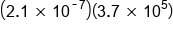Deal with numbers and 10's separately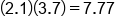Multiply numbers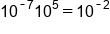Use product rule on 10's and add exponents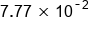Our Solution

A similar process is used to divide in scientific notation. First, we divide the decimal part of the number, and then apply a property of exponents to easily divide the powers of 10.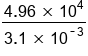Deal with numbers and 10's separately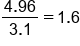Divide numbers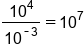Use quotient rule to subtract exponents, be careful with negatives!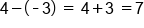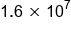Our Solution

Notice that the properties of exponents can always be used in scientific notation. This is because the exponent properties apply when the bases are the same, and the base is always 10 in scientific notation.

brainstorm
Do you think we can use any properties of exponents as shortcuts when we raise a number in scientific notation to an exponent power?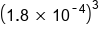Use power rule to deal with numbers and 10's separately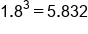Evaluate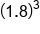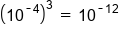Multiply exponents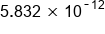Our Solution

big idea
When multiplying and dividing in scientific notation, deal with the non-exponential and exponential (containing the power of 10) separately. Multiply or divide the decimal number part first, then multiply or divide the part with exponents, applying the property of exponents to either add or subtract the exponents.
hint
Often when we multiply or divide in scientific notation the end result is not in scientific notation. We will then have to convert the front number into scientific notation and then combine the 10’s using the product property of exponents and adding the exponents. This is shown in the following examples.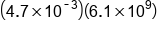Deal with numbers and 10's separately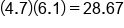Multiply numbers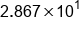Convert this number into scientific notation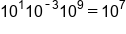Use product rule, add exponents, using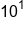from conversion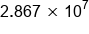Our Solution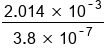Deal with numbers and 10's separately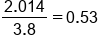Divide numbers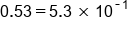Change this number to scientific notation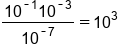Use product and quotient rule, using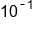from the conversion Be careful with signs: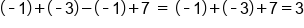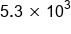Our Solution

summary
For multiplication and division in scientific notation, it is important to remember the following rules. When you're multiplying numbers that are written in scientific notation, first multiply your decimal numbers. Then, using the product of powers property, you're going to add your exponents. When you're dividing numbers in scientific notation, first divide the decimal numbers. Then use the quotient of powers property to subtract your exponents. When you're squaring a number in scientific notation, first square the decimal number. Then use the power of powers property to multiply your exponents.

Rating Header